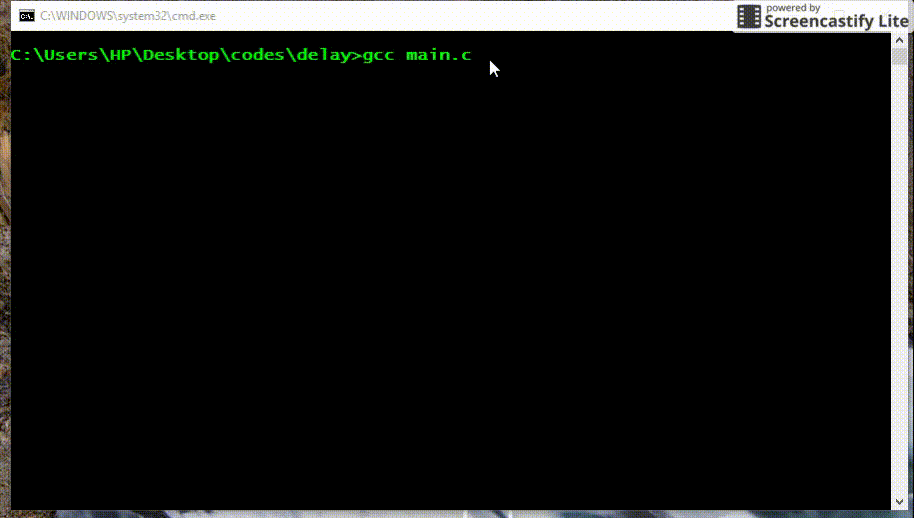# Time delay in C

• Difficulty Level : Easy
• Last Updated : 18 Dec, 2019

In this post, we will see how to give a time delay in C code. Basic idea is to get current clock and add the required delay to that clock, till current clock is less then required clock run an empty loop.

Here is implementation with a delay function.

 `// C function showing how to do time delay``#include ``// To use time library of C``#include `` ` `void` `delay(``int` `number_of_seconds)``{``    ``// Converting time into milli_seconds``    ``int` `milli_seconds = 1000 * number_of_seconds;`` ` `    ``// Storing start time``    ``clock_t` `start_time = ``clock``();`` ` `    ``// looping till required time is not achieved``    ``while` `(``clock``() < start_time + milli_seconds)``        ``;``}`` ` `// Driver code to test above function``int` `main()``{``    ``int` `i;``    ``for` `(i = 0; i < 10; i++) {``        ``// delay of one second``        ``delay(1);``        ``printf``(``"%d seconds have passed\n"``, i + 1);``    ``}``    ``return` `0;``}`

Output:

``````

This article is contributed by Pratik Chhajer. If you like GeeksforGeeks and would like to contribute, you can also write an article using contribute.geeksforgeeks.org or mail your article to contribute@geeksforgeeks.org. See your article appearing on the GeeksforGeeks main page and help other Geeks.